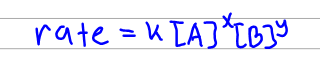# Problem: Using the given data, determine the rate constant of this reaction.A + 2B → C + DGiven Data:Trial         [A](M)           [B](M)        RATE (M/s)1              .330              .280           0.02362              .30                .560           0.02363              .660              .280           0.0944k=? the units are M-1 s-1

87% (87 ratings)
###### FREE Expert Solution

In order to find the rate constant k, we need to set up the rate law first. The problem here is that we don't know the reaction order of each reactant. Remember that the rate law only cares about the reactant and not the products. So what we have to do is to set up the rate law as shown below where x would be the order of A and y the order of B. We can then just solve for x and y later using the data given.87% (87 ratings)###### Problem Details

Using the given data, determine the rate constant of this reaction.
A + 2B → C + D

Given Data:
Trial         [A](M)           [B](M)        RATE (M/s)

1              .330              .280           0.0236

2              .30                .560           0.0236

3              .660              .280           0.0944

k=? the units are M-1 s-1

What scientific concept do you need to know in order to solve this problem?

Our tutors have indicated that to solve this problem you will need to apply the Rate Law concept. You can view video lessons to learn Rate Law. Or if you need more Rate Law practice, you can also practice Rate Law practice problems.

What is the difficulty of this problem?

Our tutors rated the difficulty ofUsing the given data, determine the rate constant of this re...as high difficulty.

How long does this problem take to solve?

Our expert Chemistry tutor, Dasha took 5 minutes and 53 seconds to solve this problem. You can follow their steps in the video explanation above.

What professor is this problem relevant for?

Based on our data, we think this problem is relevant for Professor Perrin's class at UCSD.